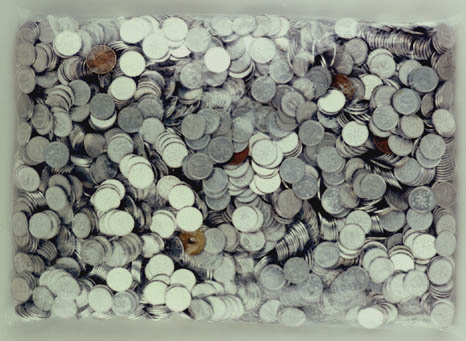to english | index | next

2620枚の硬貨から成る2924円 (金額第一四番)
2924 Yen Which Consists of 2620 Coins (Money Amount No. 14)

Japanese coins, a plastic bag
2001
photo by Takagi Shigeki

cf. 2620 and 2924 are the amicable pair which Euler found in 1747.
(Two numbers are called amicable if each equals to the sum of the divisors of the other.)
Divisors of 2620: 1 + 2 + 4 + 5 + 10 + 20 + 131 + 262 + 524 + 655 + 1310 = 2924
Divisors of 2924: 1 + 2 + 4 + 17 + 34 + 43 + 68 + 86 + 172 + 731 + 1462 = 2620